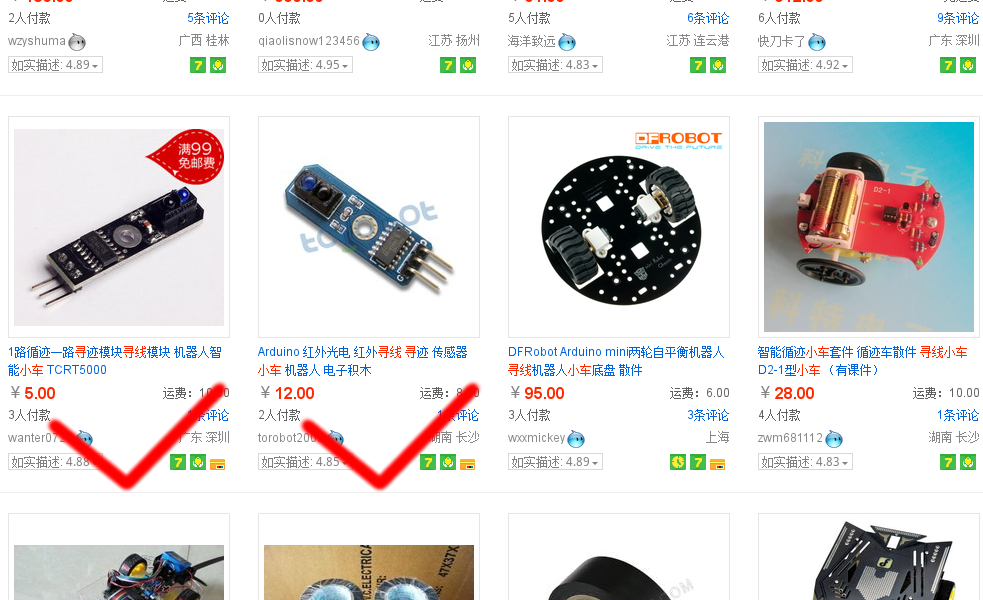# 使用键值对进行串口通讯

• 确定一条指令的开始与结尾
• 防止字节数据丢失引起的错误
• 编码与解码

```name,value;
```

```char input;
String value;
String name;

const int NAME = 1;
const int VALUE = 2;

void setup() {
Serial.begin(9600);
pinMode(13, OUTPUT);
}

void loop() {
if(Serial.available()){
process();
}
}

void process() {
if(input==','){
Serial.println(name);
}
else if(input==';'){
Serial.println(value);
run(name, value);
name = "";
value = "";
}
name+=input;
}
value+=input;
}
}

void run(String name, String value){
if(name=="speed"){
int num = value.toInt();
if(num==-1023){
digitalWrite(13, HIGH);
}
else{
digitalWrite(13, LOW);
}
}
}
```

# Arduino与Processing的串口通信

```//Processing Code
import processing.serial.*;

Serial myPort;

void setup(){
myPort = new Serial(this,"/dev/ttyACM0", 115200); //Set Serial Port

}

void draw(){
if(myPort.available()>0){
println(message);
}
}
```
```//Arduino code
int data=12345;
void setup()
{
Serial.begin(115200);//rate
}
void loop()
{
Serial.print(data); //send data
delay(1000);
}
```

```12345
123
45
12345
12345
```

Arduino和Processing的数据收发速度是不一样的。如果用Arduino延时较长时间，Processing可能读取一个字符串或字符串的一部分。如果Arduino延时较短，Processing可能读取多个字符串，但不一定完整。在读取字符串的时候，无法确定上一个字符串是否被读取了，当前字符串是否缓存完毕，因为字符串都已经切成了bytes，连成一串。这个问题是串口通信本身造成的，一定会出现。

Processing的SerialEvent事件类型就提供了这种方式，使用bufferUntil(ch)可以在遇到某个指定字符时才完成缓存。

```//Processing Code
import processing.serial.*;

Serial myPort;

void setup(){
myPort = new Serial(this,"/dev/ttyACM0", 115200);  //in fact, any rate is ok...
myPort.bufferUntil('\n');  //buffer until meet '\n', then call the event listener
}

void draw(){

}

//listen to the event. when buffer filled, run this method
void serialEvent(Serial p) {
print(inString);
}

//then the buffer will reveive all the bytes
```
```//Arduino Code
int data=12345;
void setup()
{
Serial.begin(115200);//rate
}
void loop()
{
Serial.println(data); //send data, end up with '\n'
delay(1000);
}
```

```//Processing Code
import processing.serial.*;

String message;
String temp;
Serial myPort;

void setup(){
myPort = new Serial(this,"/dev/ttyACM0", 115200); //Set Serial Port
}

void draw(){
if(myPort.available()>0){
for(int i = 0; i < temp.length(); i++){
//if meet the end mark
if(temp.charAt(i) == '\n'){
println(message);
message = "";  //clean string
}
else
message += temp.charAt(i);  //store byte
}
}
}
```
```//Arduino Code
int data=12345;
void setup()
{
Serial.begin(115200);//rate
}
void loop()
{
Serial.println(data); //send data, end up with '\n'
delay(1000);
}
```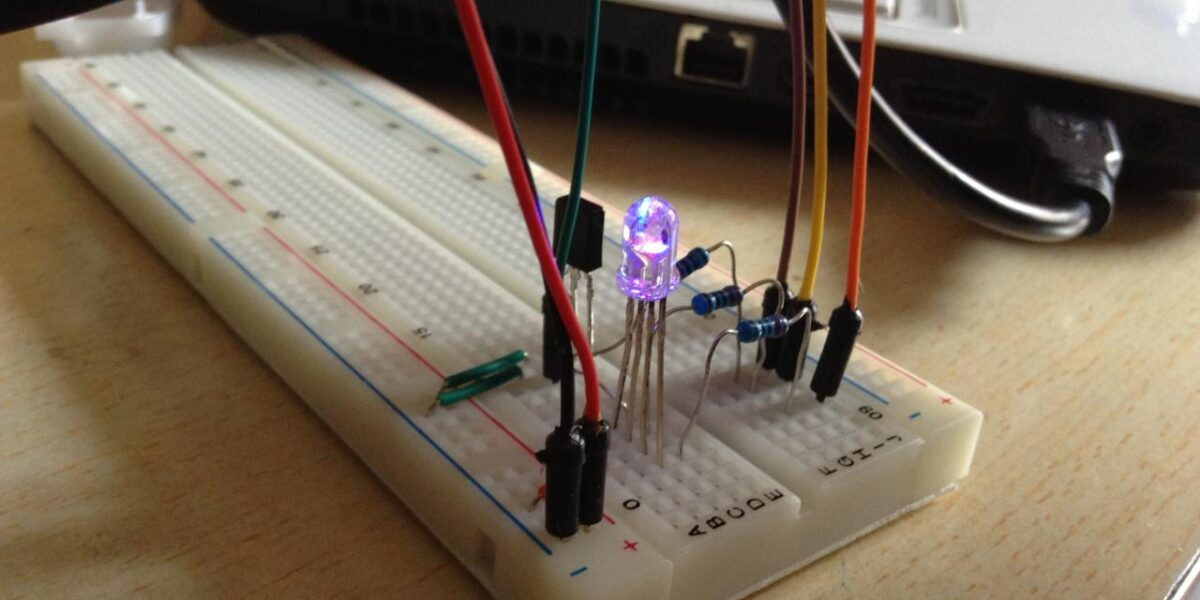# 遥控变色灯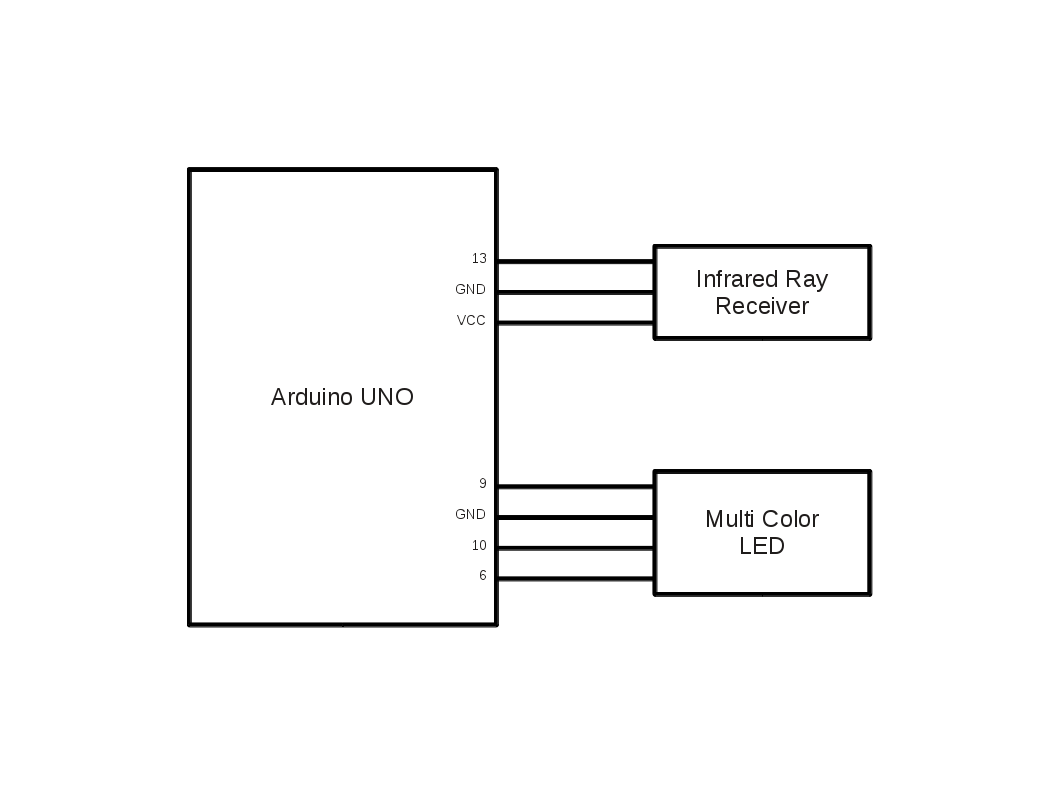Arduino有现成的红外接收库。一开始就非常顺利地接收了红外遥控的信号，并记录下了每个键所对应的值，这是通过串口发送电脑回来的。很特别的是，当一直按下一个键时，后续会重复返回FFFFFFFF。然后根据按键控制红灯的亮度，并设计成若一直按下，则会重复动作，这样就不必按很多次按钮了。

AVR单片机的6路PWM是有差异的。Arduino将其同质化处理，虽然简化了认知过程，但导致很多问题无法解释。机器人协会曾经也讨论过是否要引进Arduino，但后来还是否定了这个计划。因为Arduino的思维方式虽然在早期能够快速入门，但却导致在进阶的过程中处处遭遇困难。以后再去认识硬件细节的设计，摒弃原有的理解，是非常困难的。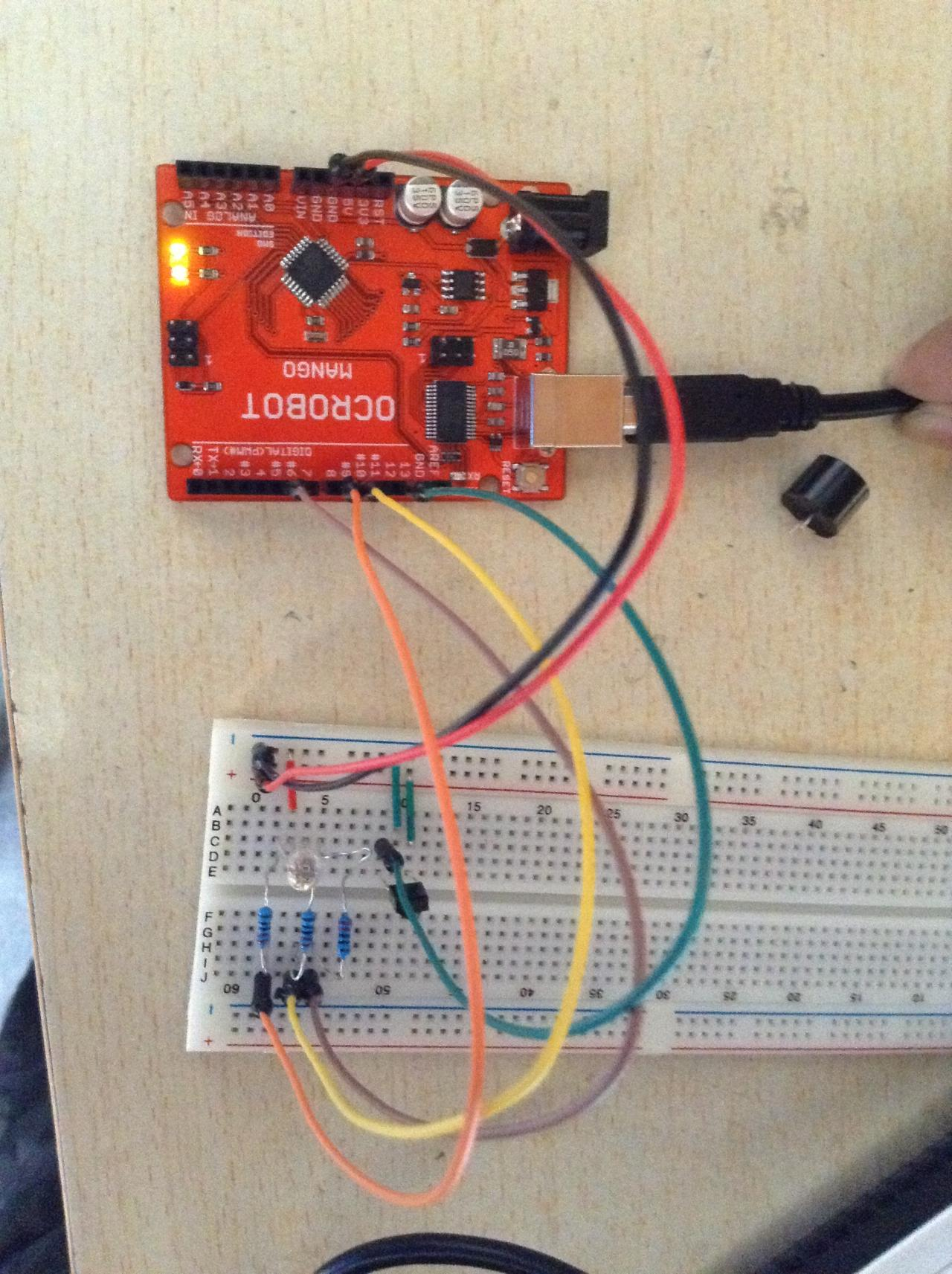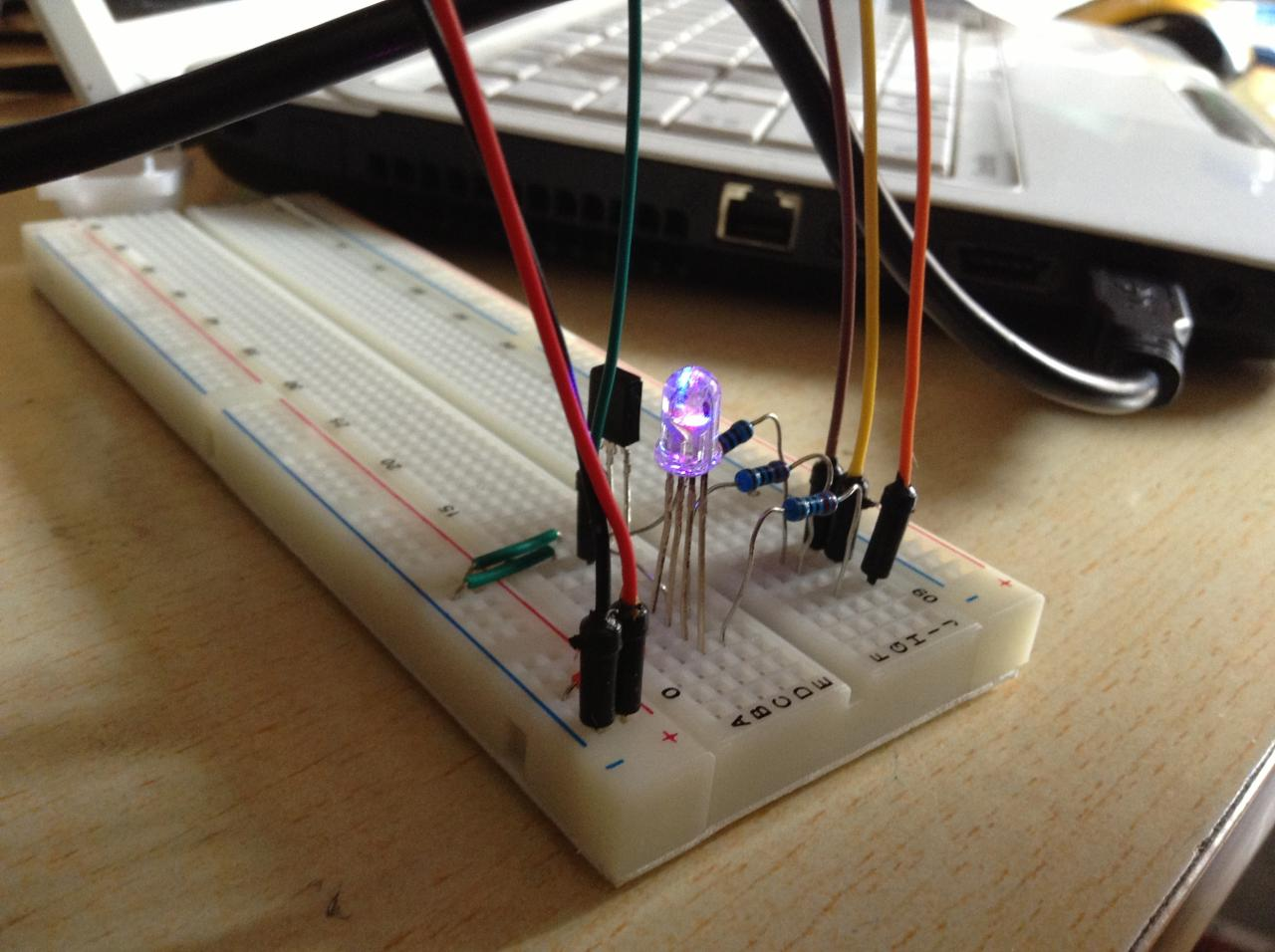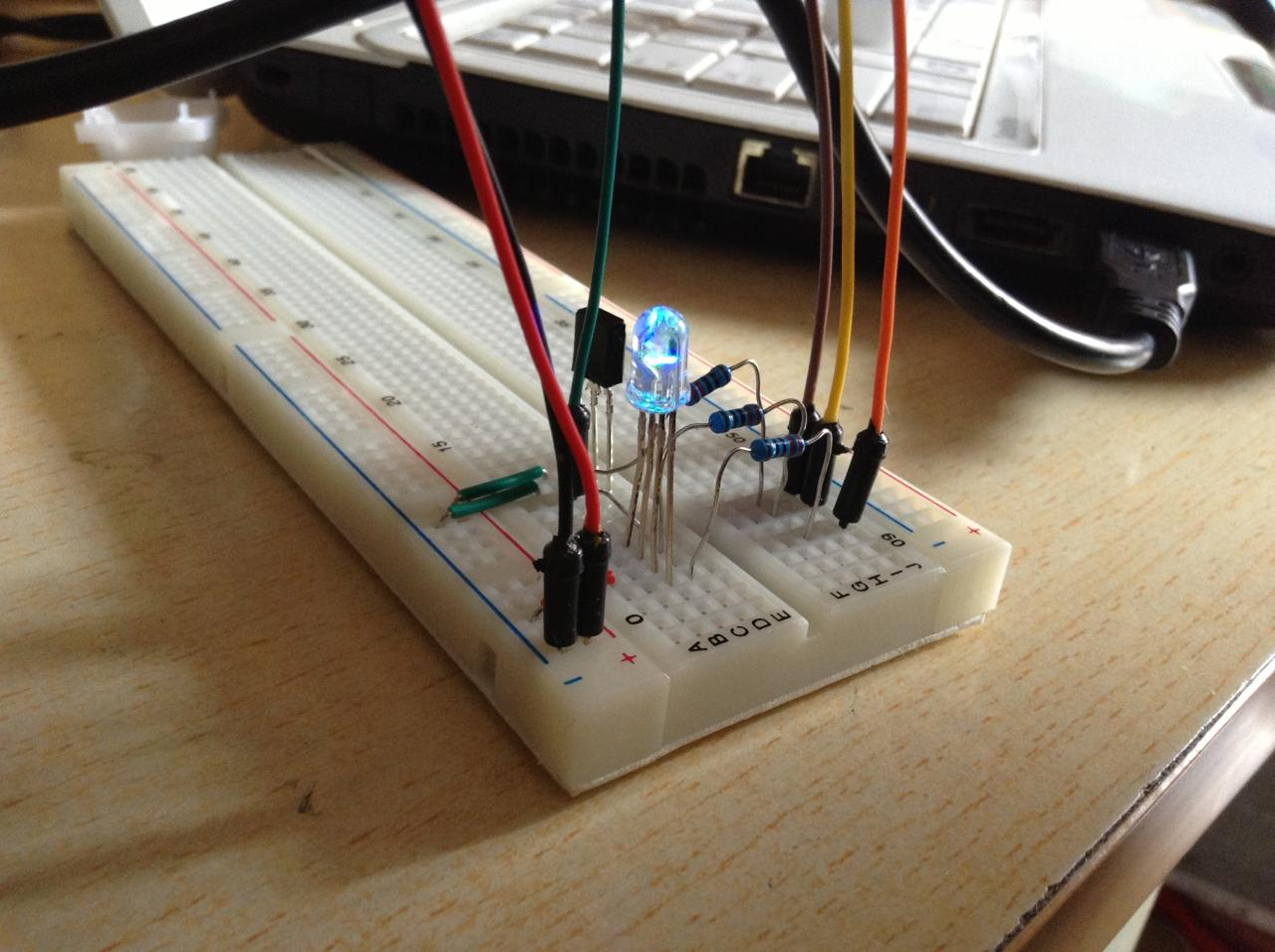```#include "IRremote.h"

int RECV_PIN = 13;

int red = 0;
int blue = 0;
int green = 0;

int last = 0;
int now = 0;

IRrecv irrecv(RECV_PIN);

decode_results results;

void setup() {
Serial.begin(9600);
irrecv.enableIRIn();
pinMode(9, OUTPUT);
pinMode(10, OUTPUT);
pinMode(6, OUTPUT);
}

void loop() {

if (irrecv.decode(&results)) {
now = results.value;
Serial.println(now);

if(now==-1)
now = last;

switch(now){
case -23971:
if(red==0)
red=255;
else
red--;
break;
case 25245:
if(red==255)
red=0;
else
red++;
break;
case 8925:
if(green==0)
green=255;
else
green--;
break;
case 765:
if(green==255)
green=0;
else
green++;
break;
case -8161:
if(blue==0)
blue=255;
else
blue--;
break;
case -22441:
if(blue==255)
blue=0;
else
blue++;
break;
case -7651:
red=0;
green=0;
blue=0;
break;
default:break;
}
irrecv.resume();
last = now;
}

color(red, green, blue);
}

void color(int r, int g, int b){
analogWrite(9, r);
analogWrite(10, g);
analogWrite(6, b);
}
```